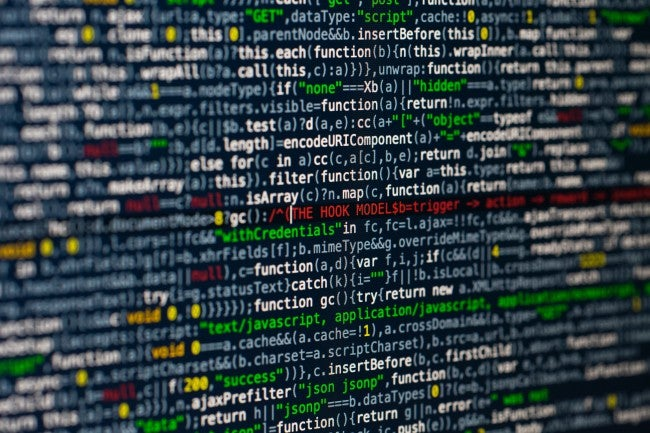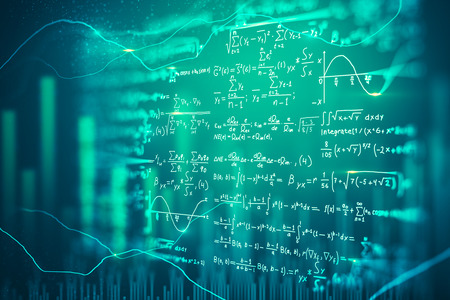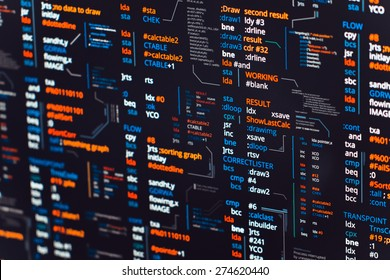Contact

# Projects

From the most stupid programs to utterly complex projects...
Here is a collection of my favourite lines of code (be them intelectually/conceptually interesting or just because I liked the overall net result).

## Here you will find

•SeeMath
Developed a companion application to Hypatia's math editor software for mathematical visualizations. Demo walkthrough of the project available here.

Explore project →

•Self Driving Car DQN
Implemented a self driving car through a Deep Q Network Reinforcement Learning Model. Tested multiple networks including a Convolutional Neural Network, Recurrent Neural Network and a hybrid model.

Explore project →

•Neural Network from Scratch
Developed an Artificial Neural Network without machine learning libraries (Tensor Flow, Keras, etc) in order to fully grasp the basic fundamentals of an artificial neural network's implementation.

Explore project →

## Apps

•SeeMath
Developed a companion application to Hypatia's math editor software for mathematical visualizations. Demo walkthrough of the project available here.

Explore project →

## Machine Learning

•Self Driving Car DQN
Implemented a self driving car through a Deep Q Network Reinforcement Learning Model. Tested multiple networks including a Convolutional Neural Network, Recurrent Neural Network and a hybrid model.

Explore project →

•Neural Network from Scratch
Developed an Artificial Neural Network without machine learning libraries (Tensor Flow, Keras, etc) in order to fully grasp the basic fundamentals of an artificial neural network's implementation.

Explore project →

•String Guesser GA
Developed a Genetic Algorithm capable of guessing the inputted string. Applied mutation to arrive at the desired string in a minimal number of iterations.
This project was an experiment for the 2020 UofT Researchathon.

Explore project →

•Linear Regression Visualizer
Animates the process of finding the line of best fit through gradient descent. Provides an intuitive visualization of the cost function and the steps taken to find the optimal minimum.

Explore project →

## Games

•Function Ride
Developed an application intended for high school students to learn more about mathematical functions and their transformations in a playful approach.

Explore project →

•Bounce Around
Simple game developed in Javascript when I was first learning to code.

Play game →

•Gaming Network
My first ever real program. I am including it here for nostalgic purposes :)

Explore project →

## HDL

•Square Animation
Manipulating VGA inputs, datapath and control systems to animate a square bouncing around on the screen

Verilog

Explore project →

•Polynomial Function Calculator
Calculates a polynomial function of the from Ax2 + Bx + C on an HDL circuit.

Verilog

Explore project →

•Sequence Detector
Finite State Machine that detects the binary sequence 1101 or four consecutive ones.

Verilog

Explore project →

•Morse Encoder
Implemented a Morse Encoder with a binary shifter and a rate divider.

Verilog

Explore project →

•Automatic Counter
Implemented a circuit that counts in hexadecimal using a rate divider.

Verilog

Explore project →

•Shifter
Implemented a binary shifter with arithmetic shift and parallel load using flip-flops.

Verilog

Explore project →

## Assembly Language

•Recursive Functions
Recursive study in MIPS (Microprocessor without Interlocked Pipeline Stages) Assembly Language.

MIPS

Explore project →

•Arrays and Indexing
Study in MIPS Assembly Language to find a specific value in the inputted array.

MIPS

Explore project →

•First Assembly Program
Script to get familiar with the language, input manipulation and Mars interface.

MIPS

Explore project →

## Data Science

•Break & Enter Crimes in Toronto
Work for the Toronto Police to analyze and find patterns in Break and Enter Crimes in the Greater Toronto Area (GTA).

R

Explore project →